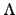# Group Homomorphisms

Definition 2.5.1   當 G, G' 是 groups 而: GG' 是從 G 映射到 G' 的函數. 如果滿足對於所有 a, bG 皆有(a . b) =(a) .(b), 則稱此函數是一個 group homomorphism.

Lemma 2.5.2   設 GG' 是 groups 且 ee' 分別為其 identity. 若是一個從 G 映到 G' 的 group homomorphism, 則:
1.(e) = e'.
2. 給定任意的 aG,(a-1) =(a)-1.

b .(e) =(e) .(e) =(e . e) =(e) = b.(a-1) .(a) =(a-1 . a) =(e) = e'.

Definition 2.5.3   若: GG' 是一個 group homomorphism, 則

im() = {(a)G' | aG}

ker() = {aG |(a) = e'},

Lemma 2.5.4   若: GG' 是一個 group homomorphism, 則 im() 是 G' 的 subgroup, 而 ker() 是 G 的 normal subgroup.(a),(b)im(), 其中 a, bG, 則利用 Lemma 2.5.2 我們知(b)-1 =(b-1). 故(a) .(b)-1 =(a) .(b-1) =(a . b-1).(a . b-1) =(a) .(b)-1 = e' . e' = e'.(g . a . g-1) =(g) .(a) .(g-1).(g . a . g-1) =(g) . e' .(g)-1 = e'.

g . a . g-1ker().Definition 2.5.5   令: GG' 是一個 group homomorphism:
1.是 onto, 則稱之為 epimorphism.
2.1-1, 則稱之為 monomorphism.
3.是 1-1 且 onto, 則稱之為 isomorphism.

Lemma 2.5.6   已知: GG' 是一個 group homomorphism, 則是一個 monomorphism 若且為若 ker() = {e}.(g1 . g2-1) =(g1) .(g2)-1 = e'.

Example 2.5.7   考慮是一個加法之下的 group, 則所有偶數所成的集合 2是其 subgroup. 考慮:2是一個 group homomorphism 定義成:(n) = 2n. 很容易看出來是一個 isomorphism. 所以2是 isomorphic.Administrator 2005-06-18